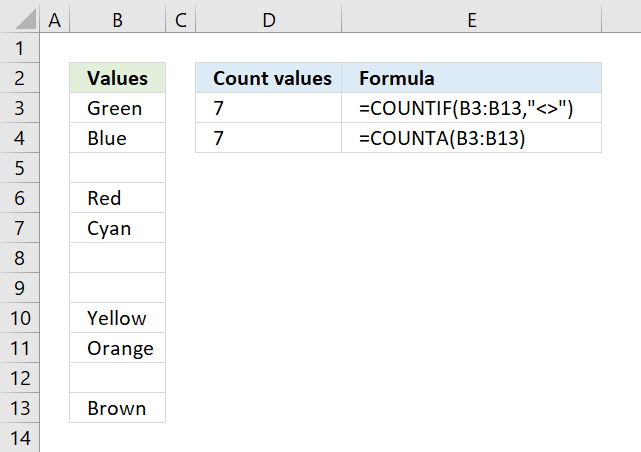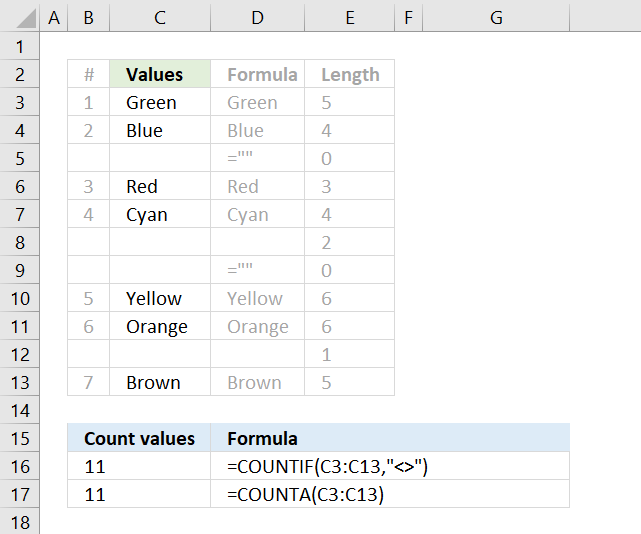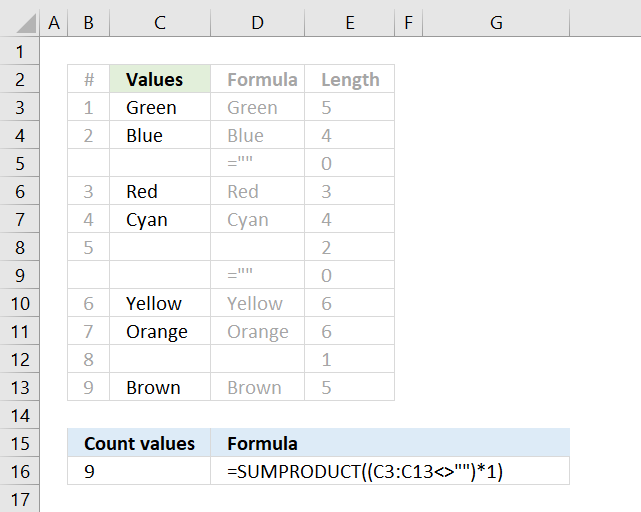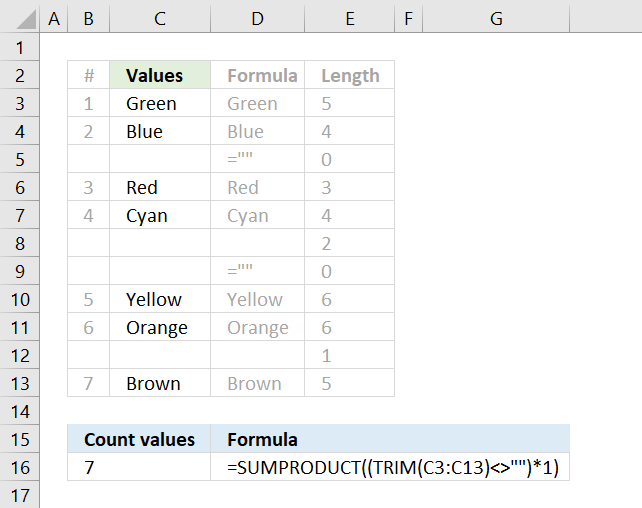Author: Oscar Cronquist Article last updated on February 04, 2019The COUNTIF function is very capable of counting non-empty values. Column B above have a few blank cells, they are in fact completely empty.

=COUNTIF(B3:B13,"<>")

The first argument in the COUNTIF function is the cell range where you want to count matching cells to a specific value, the second argument is the value you want to count.

In this case, it is "<>" meaning not equal to and then nothing, so the COUNTIF function counts the number of cells that are not equal to nothing.

The COUNTA function is even easier to use, you don't need to enter more than the cell range in one argument. The COUNTA function is designed to count non-empty cells.

=COUNTA(B3:B13)There are, however, situations where the COUNTIF and COUNTA function return unexpected results if you are not aware of how they work.

There are blank cells in column C in the picture above that look empty but they are not. Column D shows what they actually contain and column E shows the character length of the content.

Cell C5 and C9 contain a formula that returns a blank, both the COUNTIF and the COUNTA function count those cells as non-empty.

Cell C8 has two space characters and cell C12 has one space character, column E reveals their existence. The COUNTIF and the COUNTA function count those cells as non-empty as well.The following formula counts all non-empty values in cell range C3:C13 except formulas that return nothing. It checks if the values in cell range C3:C13 are not equal to nothing.

The SUMPRODUCT function can't sum boolean values so I multiply with one to create an array containing 0's (zero) and 1's. In this case, the logical expression counts cells that contain space characters but not formulas that return nothing.

=SUMPRODUCT((C3:C13<>"")*1)

becomes

SUMPRODUCT(({TRUE; TRUE; FALSE; TRUE; TRUE; TRUE; FALSE; TRUE; TRUE; TRUE; TRUE})*1)

becomes

SUMPRODUCT({1;1;0;1;1;1;0;1;1;1;1}) and returns 9 in cell B16.The formula above in cell C16 counts only non-empty values, it considers formulas that return nothing to be blank and space characters to also be blank. This is made possible by the TRIM function that removes leading and ending space characters.

=SUMPRODUCT((TRIM(C3:C13)<>"")*1)

becomes

=SUMPRODUCT(({"Green"; "Blue"; ""; "Red"; "Cyan"; ""; ""; "Yellow"; "Orange"; ""; "Brown"}<>"")*1)

becomes

=SUMPRODUCT(({TRUE; TRUE; FALSE; TRUE; TRUE; FALSE; FALSE; TRUE; TRUE; FALSE; TRUE})*1)

becomes

=SUMPRODUCT({1; 1; 0; 1; 1; 0; 0; 1; 1; 0; 1}) and returns 7.

### Download Excel file

* You will also get a weekly newsletter, unsubscribe anytime!